## Data Structures: § 2: Sets, Strings, Stacks, Heaps, and Queues

#### Instructor: M.S. Schmalz

Now that we have reviewed basic data structures, we can begin to discuss more useful (and higher-level) constructs. For example, computer scientists frequently encounter applications where it is desirable to deal with numbers in terms of sets, as one would in mathematics. Thus, we discuss sets and how they can be represented at a high level in various applications. Similarly, one can encounter problems in database or text processing applications where strings (sequences of characters) are involved. The consideration of sequences in this section, as well as lists in the previous section, leads to a discussion of data structures known as stacks and queues, which are frequently used for intermediate storage in a wide variety of practical applications. We conclude this section with a presentation and analysis of the data structure called a heap, and with its important application in sorting (heapsort).

This section is organized as follows:

2.1. Sets
2.2. Strings
2.3. String Representations of Data Structures
2.4. Stacks and Queues
2.5. Sorting with Priority Queues
2.6. Heaps and Heapsort

We begin with a discussion of sets, as follows.

### 2.1. Sets

It is often desirable to collect objects in a data structure that can be efficiently accessed, so as to be able to add or subtract objects from the collection. Several implementations of sets can provide this capability in O(log n) work per addition or deletion of an element from a set.

Recall. Set operations include union, intersection, cardinality, choice, subtraction, and complement. For purposes of this discussion, we concentrate upon union, cardinality, intersection, choice, and set subtraction, in that order.

Assumptions. Suppose we have a data structure D that allows us to perform random access insertion and deletion operations in O(log n) work per operation. This is not a fanciful assumption, because such structuresd exist as discussed in Section 2.6.

For purposes of simplicity in this discussion, assume that the work incurred by insertion or deletion is not dependent on the type of data stored in D. Also assume that each set representation S based on D has a counter associated with it, called size(S), which contains the number of elements in S.

In the following definitions, we also assume that we have two sets A and B, whose representations are based on D.

• Set Union. Recall that set union is defined as follows:

A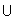B = {x : xA or xB} .

Given this definition, the following procedure can be employed to compute the union of two sets A and B, putting the result into B:

```        Union(A,B: set)
{ for all x in A
if x is not in B
then insert x into B
endfor
}
```

Analysis. If determining whether or not an element x is in B requires logarithmic work, then the preceding code takes O(|A| · log(|B|)) work, since we must determine for each element of A whether or not that element is in B.

• Set Intersection. Recall that set intersection is defined as follows:

A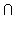B = {x : xA and xB} .

Given this definition, the following procedure can be employed to compute the intersection of two sets A and B, putting the result into another set C:

```        Intersection(A,B,C: set)
{ for all x in A
if x is in B
then insert x into C
endfor
}
```

Analysis. If determining whether or not an element x is in B requires logarithmic work, then the preceding code takes O(|A| · log(|B|)) work, since we must determine for each element of A whether or not that element is in B. The insertion cost is also logarithmic in the number of elements that are in both A and B, but does not predominate in the Big-Oh complexity estimate.

• Cardinality. Recall that set cardinality is the number of elements in a set. Thus, we need only count the elements of a set to determine its cardinality. Since we assumed that D has a counter c associated with it, we simply output the counter, as follows:

```        Cardinality(A: set)
{ return(size(A))
}
```

Analysis. Since we already have the set cardinality stored in the variable size(A), this pseudocode requires O(1) work.

• Set Subtraction. Recall that set subtraction is defined as follows:

A \ B = {x : xA and x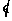B} .

Given this definition, the following procedure can be employed to compute the subtraction of two sets A and B, putting the result into another set C:

```        Subtraction(A,B,C: set)
{ for all x in A
if x is not in B
then insert x into C
endfor
}
```

It is easy to see that this pseudocode for set subtraction is a simple modification of the code for set intersection given previously.

Analysis. If determining whether or not an element x is in B requires logarithmic work, then the preceding code takes O(|A| · log(|B|)) work, as did the union and intersection operations.

• Choice. Recall that choosing an element from a set A via the choice operation returns an element selected at random from A. In order to produce this effect, we need to assume that the set is somehow indexed, that is, each element of the set has associated with it a unique object. For example, one can index a set with the positive integers. We would express this as A[i}, i = 1..n .

Given this definition, the following procedure can be employed to obtain an element at random from a set:

```        Choice(A: set)
{ n = size(A)
i = random integer between 1 and n
return(A[i])
}
```

Analysis. The primary cost in the choice operation is the generation of a random number. If this is done using a lookup table, then the cost tends to be quite small (I/O operations only). Otherwise, arithmetic operations can be involved, and the cost would thus increase.

• Remark. We have thus far not discussed the operation that is basic to each of the preceding algorithms, namely, determining if an element is in a set. This is called set membership. For example, if the data structure D upon which each set is based is an object called a search tree, then we can implement a full query of the set in O(log n) work. So, let us assume that we have some function called member(x,S) that determines if an element x is in a set S, and returns a Boolean true value if this is so (and returns false otherwise).

We can then rewrite most of the previously-given pseudocode in terms of the membership function. For example, here follows the code for set intersection expressed in terms of the membership operation on indexed sets A and B:

```        Intersection(A,B,C: indexed set)
{ nA = Cardinality(A)
nB = Cardinality(B)
if nA > nB then
for i = 1 to nB
if member(B[i],A)
then
size(C)    = size(C) + 1
C[size(C)] = B[i]
endif
endfor
else
for i = 1 to nA
if member(A[i],B)
then
size(C)    = size(C) + 1
C[size(C)] = A[i]
endif
endfor
endif
}
```

It is easy to see that this pseudocode is much bulkier, due to the implementational assumptions of (a) membership function, and (b) indexed set. However, this expression of code has allowed us to determine that the insert function on an indexed set could be expressed quite simply, for example:

```	         size(C)    = size(C) + 1
C[size(C)] = A[i]
```

describes insertion of A[i] into set C. (Notice that this is similar in concept to an array I/O operation.)

We will revisit these concepts of sets, search trees, and indexing occasionally, especially when we discuss heaps.

• We next move on to the string data structure.

### 2.2. Strings

We have all used text processing programs such as Microsoft Word, Word Perfect, or PageMaker to prepare documents, search existing documents for prespecified words or phrases. However, we often fail to recognize that this type of effort involves the use of a very specific type of data structure, called a string. In this section, we examine the definition of a string, as well as operations over strings and their complexity.

• Definition. A string is a sequence of characters taken from an alphabet A.

Example. Given the alphabet A = {a,b,d,y}, one can construct the string a bad baby.

Example. Given the modern English alphabet A = {a,b,...,z,A,B,...Z}, one can view this sentence as a string (inclusive of punctuation such as period, space, and comma, as well as delimiters such as parentheses, braces, and brackets).

Operations on Strings. Strings can be manipulated by operations such as concatenation, length, substring and pattern recognition. We discuss each of these operations as follows.

• Definition. Given two strings s and t, the concatenation operation, written as s || t or s + t, appends t to s, such that the last character of s is followed by the first character of t and the remainder of t.

Example. Given s = "hello" and t = "dave" s + t = "hellodave".

Algorithm. Given two strings s and t of length M and N, respectively, the concatenation operation s + t can be expressed in pseudocode, as follows:

```     concatenate(s,t : string):
{ for i = 1 to M do           # M iterations
output(s[i])              # 1 I/O per iteration
for i = 1 to N do           # N iterations
output(t[i])              # 1 I/O per iteration
}
```

Analysis. The preceding algorithm requires O(M + N) I/O operations, due to the two non-nested loops.

• Definition. Given a string s, the length operation returns the number of characters in s.

Example. Given s = "hello", length(s) = 5.

Example. Given s = "hello world", length(s) = 11, since leading blank symbols and internal blank symbols are included in the length calculation.

Algorithm. Given a string s, and assuming that s terminates with a null character, the length of s can be computed as follows:

```     length(s : string):
{ L = 0
repeat until s[i] = null
L = L + 1
return(L)
}
```

Analysis. The preceding algorithm requires N incrementations to compute the length of an N-character string.

• Definition. Given a string s of length N, a substring of s is a contiguous partition of s that has length less than or equal to N.

Example. Given s = "friday", the substring s[3:4] = "id", and the substring s[4:6] = "day", as shown below.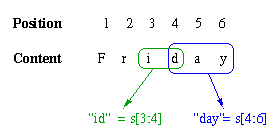Figure 2.2.1. Example of substring extraction.

Algorithm. Given a string s, the [i:j]-th substring of s can be computed as follows:

```     substring(s : string, i,j: integer):
{ for k = i to j do
output(s[k])
}
```

Analysis. The preceding algorithm requires O(j - i) I/O operations to compute the [i:j]-th substring. If i > N or j > N or i < 1 or j < 1, then the substring is undefined.

• Definition. Given a string s of length N, and a test pattern t of length M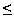N, if t is a contiguous partition of s, then t is said to be found in s.

Example. Given s = "friday", the pattern "id" is the [3:4]-th substring of s, and is thus found in s.

Algorithm. Given a string s of length N, and a test pattern t of length MN, the process of locating t in s can be computed many different ways. Here is one such expression in pseudocode, which is also called template matching or brute-force pattern matching:

```     find(s,t : string)
{ M = length(t)
for i = 1 to (length(s) - M + 1)
{ j = i+M-1]
if s[i:j] = t[1:M] then return(i) }
return(-1)
}
```

Analysis. The preceding algorithm requires O(N2) comparisons to implement brute-force pattern matching. Since t = s is possible, we say that length(t) = O(length(s)) = O(N). Since there are N - M + 1 iterations of the loop in the preceding algorithm, this implies O(N2) comparisons.

• ### 2.3. String Representations of Data Structures

It is sometimes useful to print out a data structure in ASCII form, for portability of structural and value-specific information. There are many different representations of data structures that can be used for this purpose. Here follow a few such string representations.

List -- Given a list L = (a,c,1,3,b,4,e,r,2,6), one can represent L using the following string:

` [LIST L [a c 1 3 b 4 e r 2 6]]`

Here, the datatype string LIST tells us what type of data structure is encoded, L denotes the name of the list, and the elements are space-delimited within enclosing brackets.

Vector, Set -- A vector (1-D array) or set can be represented in the same manner as a list, using the type identifier VECTOR or SET instead of LIST.

Matrix -- A matrix is a 2-D array. For example, a 2x3-element matrix A, given by

```    [  1 2 3  ]
[  4 5 6  ]
```

can be represented in terms of the string

` [MATRIX A [2 3] [1 2 3 4 5 6]]` ,

where the two numbers following the name A denote the number of rows and columns in A, and the values of A are listed in row-major scanning order. The other common type of scanning order, called column-major, would list the values of A as ```1 4 2 5 3 6```.

Multidimensional Array -- A multidimensional array would be encoded similarly to a 2-D array or matrix. The sizes of each array dimension would be listed after the array name, and the elements would be listed in some predetermined scanning order (customarily row-major).

As we introduce other data structures and their operations, we will include the string representation of each salient data structure.

### 2.4. Stacks and Queues

Various types of dynamic storage are employed by system and applications software. For example, a compiler frequently uses stacks to store information that is retrieved soon after it is stored. Multiprocess computing and network management software often employs queues for managing waiting and executing jobs (programs submitted by users).

Concept. A stack implements a last-in, first-out (LIFO) buffer.

Definition. A stack is a list where insertion and deletion operations are performed only at the head of the list.

Observation. A stack can be thought of like the spring-loaded stacks of plates in a cafeteria. You put a plate (data object) on top of the stack, and all you can see is the top plate. When you remove a plate from the stack, you still see the top plate only, unless the stack is empty.

Size - returns the number of objects on (currently stored in) the stack, an integer.

IsFull,IsEmpty - returns true if the stack is full (size equals some maximum constraint), or empty (size equals zero), respectively.

Push - adds an object to the top of the stack if the stack is not full.

Pop - removes an object from the top of the stack if the stack is not empty.

Peek or Top - returns a copy of the object on top of the stack if the stack is not empty.

Example. Let a stack S begin as empty, then let us push 1 onto the stack. This is shown in Step 1 of Figure 2.4.1. Next, we push the values 2 and 3 onto the stack, as shown in Steps 2 and 3. If S has capacity n = 3, then S is full. Popping the values off the stack as shown in Steps 4-6 yields size = 0 (empty stack). Note how the values that were first pushed onto the stack come off the top of the stack last. That is why the stack is called a LIFO buffer.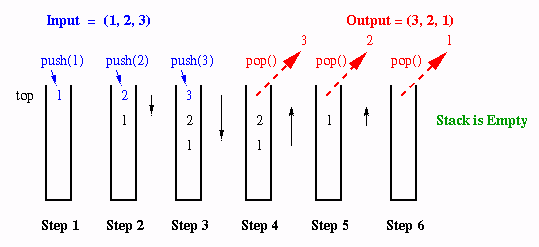Figure 2.4.1. Stack operations that push a sequence (1,2,3) onto a stack, then pop it off to yield output (3,2,1).

Implementation. A stack is usually implemented with a list, but can be implemented with an array. The list implementation was previously described at a high level. Note that push and pop operations are implemented at the head of the list. Additionally, the implementation should maintain a counter for the list size that is incremented when a push operation is executed and decremented when a pop operation is executed. A peek operation simply copies the value at the head of the list. This can be implemented with pop, copy, and push operations.

The array implementation of a stack is more interesting, and can be faster on many different types of machines. The array implementation has a counter called a stack pointer that denotes the position of the array where a value was last inserted. Assuming that the array a is one-dimensional and has indices ranging from 1 to some large value N, let the stackpointer be denoted by j. This means that the last push() operation stored value a[j].

If another push operation is specified, then we need to perform the following steps:

1. Check to see if i = N. If so, the stack is full and we cannot execute push() and must notify the user of stack overflow.

2. If j < N, then increment j and store the value v in push(v) as a[j] = v.

In contrast, if a pop operation is specified, then we need to perform the following steps:

1. Check to see if j > 0. If not, the stack is empty and we cannot execute pop() and must notify the user of stack underflow.

2. If j > 0, then decrement j and return the value v at a[j].

3. As an optional step, store a sentinel value that indicates a null value in a[j]. This is useful in case the stackpointer is lost, corrupted, or destroyed.

The peek operation is implemented as return(a).Figure 2.4.2. Stack operations when a stack is implemented in terms of an array.

Concept. A queue implements a first-in, first-out (FIFO) buffer.

Definition. A queue is a list where insertion (deletion) is performed only at the tail (head) of the list.

Observation. A queue can be thought of like the line of customers in the bank or post office. When a customer arrives in the queue, that person is added to back (tail) of the queue. When the clerk calls for the next customer to be served, that person exits the queue at the front of the queue.

Size - returns the number of objects in the queue, which is an integer.

IsFull,IsEmpty - returns true if the queue is full (size equals some maximum constraint), or empty (size equals zero), respectively.

Enqueue - adds an object to the rear of the queue if the queue is not full.

Dequeue - removes an object from the front of the queue if the queue is not empty.

Front - returns a copy of the object at the front of a nonempty queue.

Example. Let a queue Q store the elements 1, 2, and 3, where 1 is at the front of the queue. This is shown in Figure 2.4.3, Steps 1-3, below. If Q has finite capacity, then we can detect that Q is full. Dequeueing of the values 1, 2, and 3 is shown in Figure 2.4.3, Steps 4-6, which yields an empty queue. Note how the values that were first enqueued come out the front of the queue first. That is why a queue implements a FIFO buffer.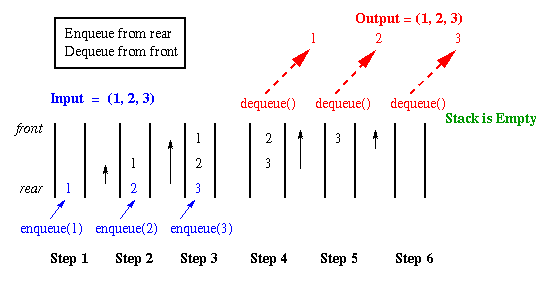Figure 2.4.3. Queue operations that enqueue each element of a sequence (1,2,3), then dequeue to yield output (1,2,3).

Implementation. A queue is usually implemented with a list, since array implementations are costly. For example, note that in Figure 2.4.3, Steps 4-6, the contents of the queue would have to be shifted up one location at each dequeueing operation. Alternatively, one could use front and rear pointers to denote the limits of the queue, but the contents would periodically have to be shifted, so that the front of the queue would be able to accept data. This clearly requires O(n) work for an n-element queue. Hence, array implementations of queues are not customary.

We next examine a special type of queue, called a priority queue, which allows us to perform special types of operations, among them sorting of values in the queue.

### 2.5. Sorting with Priority Queues

Let us assume that we have a queue with the special property that the smallest value in the queue is always at the front of the queue. Don't worry just yet about how that would be implemented; let's simply assume that property exists. Given such a queue, sorting the sequence of numbers stored in the queue would be easy, if we wanted to sort in ascending order. For example, for the i-th of n entries in the queue, we would delete the front of the queue, and put it in the i-th position in the sorted output.

Special types of queues like this exist, and they are called priority queues. We discuss this concept in detail, as follows.

Concept. A priority queue implements queue-based storage of objects, where each object is associated with a priority value. The higher priorities indicate objects that have greater operational importance.

Definition. A priority queue is a queue, where each element of the queue stores a tuple containing a value x and a priority p(x) that is an integer.

Application. Priority queues are often used in scheduling applications, where a given job or work order is assigned a priority. For example, if "1" denotes the highest priority, then one would want to perform the highest priority jobs first (the elements of the queue where p(x) is smallest).Figure 2.5.1. Priority queue that stores {(B,3), (A,1), (C,4), (D,2)}, where the second element of each tuple denotes the priority, with 1 being the highest priority.

Implementation. Priority queues are usually implemented using the heap data structure (discussed below). The heap ADT operation of MIN (or MAX) allows one to find the minimum- or maximum-valued element stored in a heap that stores n data, in O(log n) time. Thus, if the MIN or MAX operation acts on the priority value, then one could (in the previous discussion of applications) retrieve the smallest priority value, which would be associated with the heap (or priority queue) element storing a pointer to the highest-priority job.

Various instances of priority queue are used in communications (e.g., network traffic management), operations research (e.g., assembly line optimization), and job scheduling (e.g., for a multiprocessor computing machine), among other applications. Thus, priority queues are of practical importance.

### 2.6. Heaps and Heapsort

We have overviewed the priority queue and some of its applications, and have stated that a priority queue can be implemented in terms of a heap. In this section, we discuss the heap ADT, operations upon a heap, and the special algorithm called heapsort, which allows us to sort a sequence of numbers S using a heap representation of S.

Concept. The heap is a data structure based on the structure of a binary tree. A max-heap has the maximum value of a sequence stored in the heap located at the top of the heap (root of the binary tree). A min-heap has the minimum of the sequence located at the top of the heap.

Definition. A heap is a binary tree that stores distinct values, and has the following properties:

Heap Structural Property - The heap must be a complete binary tree. That is, each level of the tree except for the bottom level must be completely full. The bottom level must have all its leaves moved to the left, with any blank leaves located to the right of that level.

Heap Order Property - Since the heap must be a binary tree, each internal node (except the ones on the second-to-lowest level) has two children. Only one node on the second-to-lowest level of the tree may have one child, and that is the node on the lowest level of the tree.

In a max-heap, each parent node (including the root) has a value (often called the key of that node) that is larger than the keys of its children. Additionally, in a max-heap the left-child of the parent should have a key that is larger than the key of the right-child.

In a min-heap, each parent node (including the root) has a value (often called the key of that node) that is smaller than the keys of its children. Additionally, in a min-heap the left-child of the parent should have a key that is smaller than the key of the right-child.

Example. Suppose we have a sequence S = {1,2,3,4,5,6}. Let H be a min-heap that stores S, as follows: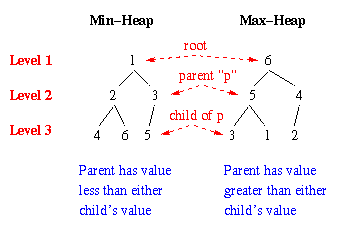Figure 2.6.1. Min-heap and Max-heap that store {1,2,3,4,5,6}, where landmark features of each heap are shown in red.

Heap Structural Property Satisfied. Observe that the preceding heaps are complete binary trees, since (a) each parent has two children,(b) all levels except the lowest level (Level 3) are full, and (c) all the elements of Level 3 are pushed to the left, with the blank element being to the right of the nonblank elements. In the min-heap, note that the value 5 is the only child of the rightmost node of the next-to-lowest level (Level 2).

Heap Order Property Satisfied. Observe that the preceding min-heap has each parent's key (or value) smaller than the keys of its children. For example, 1 is the parent of 2 and 3, 2 is the parent of 4 and 6, and 3 is the parent of 5. Also note that the left-child of each internal node is smaller than the right-child. For example, 2 < 3 and 4 < 6. The converse of the inequality holds for the max-heap.

It is important to note that the sequence stored in the heap need not be in strictly increasing or decreasing order. The heap order property does not specify this. Thus, we can have 2 as the parent of 4 and 6, rather than 4 and 5.

Observation. If a data structure stores distinct values and satisfies the heap structure and order properties, then it is a heap. Otherwise, it is not a heap.

Example. The following data structures are not heaps: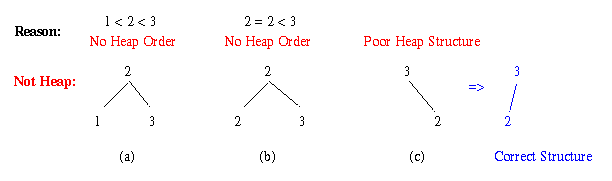Figure 2.6.2. Three tree structures that are not heaps: (a,b) both children are not less than or greater than parent, and (c) right-child occupied instead of left-child (corrective rotation shown in blue).

In Figure 2.6.2, a) is not a heap because the parent key 2 is less than its left-child's key, but greater than its right-child's key. This means that it is neither a min-heap or a max-heap. Example b) is not a heap because it stores indistinct values, and thus violates the heap order property. Example c) is not a heap because it is not a complete binary tree. However, c) could be rotated so that 2 would be the left-child of 3. In that case, it would be a complete binary tree.

Heap ADT Operations. The following operations are performed on a min-heap or max-heap H:

Min(H) (or Max) returns the root key of a min- (max-)heap.

Delete(H) removes the root key of a min- (max-)heap, and manipulates the values in the heap to restore the heap structure and order properties.

Insert(H) inserts a new value in the heap, after testing to see if that value is already in the heap (in which case it is replaced at its existing position, not duplicated in the heap).

Size(H) determines how many values are in the heap.

IsEmpty(H) queries Size(H) equal zero.

IsFull(H) determines if Size(H) equals some prespecified maximum. This is useful primarily in array implementations of heaps (discussed below).

#### 2.6.1. Implementation of Heap ADT Operations.

Now that we have studied the various heap ADT operations, we will review the tree- and array-based implementations of a heap. The tree-based implementation has the advantage of expandability (in principle, has no fixed size), but requires relatively high maintenance overhead. In contrast, the array-based implementation has low maintenance overhead but is limited by fixed size.

Concept. A tree structure can be used to represent a heap, since the heap is a binary tree. A typical binary tree node has (1) a slot for a pointer to the the parent node, (2) two slots for a pointer to each child node, (3) one or more slots for value nodes, and (4) optional slots for flags (e.g., that are used in tree traversal algorithms to be studied later in this course).

Example. A simple tree node suitable for heap element implementation is shown in Figure 2.6.3(a). The node has a parent pointer, two child pointers, and one slot each for a value x and a priority p(x) to implement a priority queue. Figure 2.6.3(b) shows how these nodes would be connected together and their values assigned to store the elements of {(A,3),(B,4),(D,2),(C,1)} in a heap.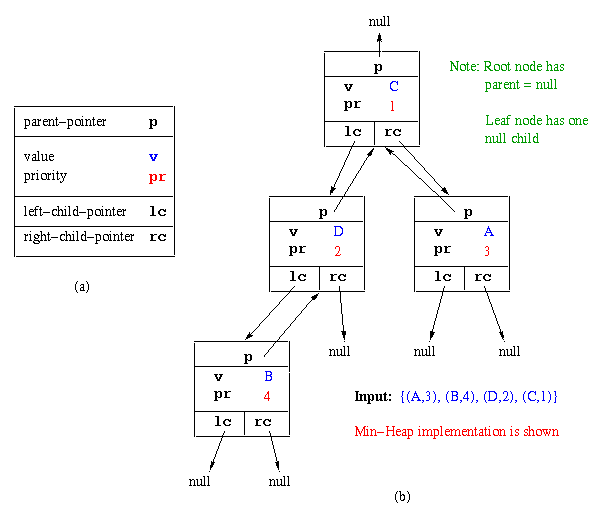Figure 2.6.3. Tree structure for simple heap implementation: (a) Single heap element, (b) Elements of {(A,3),(B,4),(D,2),(C,1)} stored in a heap using the heap element in a).

There are several ways to describe the operations that are constitutent to inserting or deleting values from a heap. It is usually easier and more straightforward to explain the functionality of these operations if we first understand the array implementation of the heap data structure, which is described as follows.

Observation.Recall that a heap is a complete binary tree (heap structure property). Thus, the root node (Level 1) consists of one heap element, while the i-th level of the tree that implements a heap contains 2i-1 elements. Since each level of the tree represents a contiguous partition of the heap scanned in breadth-first order, it is possible to concatenate these partitions to form a linear data structure such as an array or list. This representation is shown in Figure 2.6.4 for the input sequence S = {1,6,3,8,5,4}, which is stored in a heap.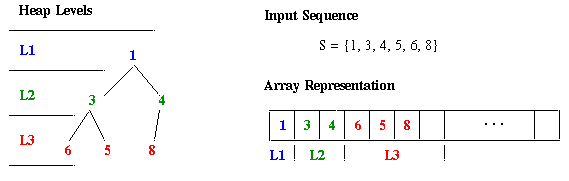Figure 2.6.4. Array implementation of (a) Heap constructed from sequence S = {1,6,3,8,5,4}, and (b) Array implementation of a).

Remark.It follows from the previous observation that an array representation a of a heap can be constructed by letting values a contain Level 1 of heap, a through a contain Level 2 of the heap, and a[2(i-1)] through a[2i-1] contain Level i of the heap.

The advantage of an array-based heap representation is that insertion can be performed at the last non-null element of the array. For example, in Figure 2.6.4, the next insertion would be performed after the value "8". We call this insertion location the last node, because it is the last node in the array representation of the heap that can be filled, while still retaining the heap structure property.

The chief disadvantage of representing a heap using an array is that when the root value (located at a) is deleted, then one must move all the values in the array. A similar situation occurs when a value is inserted into or deleted from the heap, and values in the heap must be shuffled or reordered to preserve the heap-structure and heap-order properties.

We next show how the various Heap ADT operations can be implemented in terms of the heap element shown in Figure 2.6.3. We begin with the more easily-implemented operations of Min(), Size(), IsEmpty(), and IsFull().

Min(H) (or Max) returns the root key of a min- (max-)heap. For example, if the heap is implemented as an array a (per Figure 2.6.4), then the root key is in the heap element a(1). If the heap is implemented using a tree data structure (per Figure 2.6.3), then (a) one always keeps a pointer r to the root, and (b) one accesses `r.v`, as shown in Figure 2.6.3, to get to the root key.

Size(H) determines how many values are in the heap. Recall how we kept count of the number of elements in a list using an integer variable `count`. The same technique is used in implementing Size() for a heap. That is, if a call to Insert() (or Delete()) is executed, then `count` is incremented (decremented). To find the size of the heap, a call to Size() merely returns the value of `count`.

IsEmpty(H) checks to see if there are any values in the heap. To implement this operation, call Size(H), then check the value returned by Size to see if it is zero.

IsFull(H) determines if Size(H) equals some prespecified maximum. This is useful primarily in array array implementations of heaps, and in tree implementations on small processors with limited memory.

The Insert and Delete operations are more involved, because they modify the heap and thus require checking of the heap structure and heap order properties. This checking cannot be efficiently performed across the entire heap. Instead, the insertion or deletion is performed on a subtree of the heap, to keep the cost to O(log n) operations.

In the following explanations and pseudo-code, we assume that a max-heap is implemented in a one-dimensional array called heap. Further assume that the heap has been built and that its last node (the one most recently inserted) is at `heap[size]`. That is, the number of elements in the heap is denoted by `size`.

Insert(H) inserts a new value in the heap, after testing to see if that value is already in the heap (in which case it is replaced at its existing position, not duplicated in the heap). In the following pseudocode, assume that the value `x` to be inserted in the max-heap has been determined to be unique.

```  Insert(x,size : integer; heap : array[1:n])
{  i       = size + 1                     # set i to be after last node
while( i != 1 and heap[i/2] < x ) do   # preserve heap order property
{ heap[i] = heap[i/2]                  # oops, we have to swap x with
i       = i/2                        #   its parent by doing the first
}                                      #   part of the swap, then reset i
heap[i] = x                            # put x in heap[i] to complete the
}                                         #   insertion procedure
```

Delete(H) removes the root key of a min- (max-)heap, and manipulates the values in the heap to restore the heap structure and order properties. Assume that the key to be removed is denoted by `x`. The procedure listed below crawls down through the heap reordering the elements (test element is called `y`) to preserve the heap properties. This is done in O(log n) comparison operations.

```  Delete(x,size : integer; heap : array[1:n])
{  if (size == 0) then return null        # oops, heap is empty

x    = heap                         # get root of non-empty heap

y    = heap[size]                      # start restructuring w/ last node
size = size - 1                        # shrink heap by 1 since x gone
i    = 1                               # let's start at the root
ci   = 2                               #   and test the child of heap

while (ci <= size) do                  # heap traversal loop w/ ovfl test
{ if (ci < size                        # again, check heap size
and heap[ci] < heap[ci+1]) then  # check heap order property
ci      = ci + 1;                  # crawl to next node in BFS order

if (y >= heap[ci]) then              # can we put y in heap[i]?
+-<---  exit while loop                    # yes, heap order is preserved
|     else                                 # no, swap heap[i] with child:
|       heap[i] = heap[ci]                 #   move child up
v       i       = ci                       #   go down one level
|       ci      = 2 * ci                   #   set ci to child node of i
|   }
+-> heap[i] = y                            # put y in its proper place in heap

return(x)                              # one last thing - return the
}                                         #   root value x to end deletion
```

The preceding algorithm is quite elegant, because it uses very simple comparison operations to test whether the heap structure and order properties are preserved. In the best case, only one swap (one comparison and two I/O operations) needs to be made. In the worst case, the heap is ordered opposite of the order needed for insertion of y, and all nodes of the heap must be reordered, leading to a total overhead of O(log n) comparison operations and a corresponding number of I/O operations.

The tree-based implementation of heap ADT operations will be assigned as part of a class project, and is discussed in Chapter 9 of Dr. Sahni's book.

#### 2.6.2. HeapSort Algorithm.

Using the Heap ADT operations defined in Section 2.6.1, one can construct a simple heap-based sorting algorithm that operates in O(n log n) time.

Concept.The HeapSort algorithm (1) forms a heap using the INSERT operation, then (2) unloads the heap by removing the root note using the DELETE operation. The INSERT operation, described in Section 2.6.1, preserves the heap-structure and heap-order properties during heap formation. The DELETE operation, also described in Section 2.6.1, preserves the heap-structure and heap-order properties at each application of the operation. Thus, we are certain that the root value of the heap is the minimum of the set of integers stored in the heap each time the MIN or DELETE operation is called.

Algorithm. Given an input sequence, which we assume is stored in an n-element array of integers, denoted by a, HeapSort proceeds as follows:

```   BuildHeap(a : array [0..n] of int; H : heap)
{ int i,n
for i = 1 to n do                    # n iterations of the loop
INSERT(a[i],H)                     # O(log n) work per iteration
}                                      # Total Work = O(n log n)

HeapSort(H : heap; a,b : array [0..n] of int)
{ int i,n
BuildHeap(a,H)                       # O(n log n) comparisons
for i = 1 to n do                    # n iterations of the loop
{ b[i] = MIN(H)                      # O(1) I/O operation
DELETE(H) }                        # O(n log n) comparisons
}                                      # Total Work = O(n log n)
```

where b denotes the sorted array. In this case, we assume a min-heap, so we can sort in ascending order. A max-heap would be used for sorting in descending order.

Complexity. The HeapSort algorithm incurs O(log n) operations (comparisons and I/O operations) for each insertion into an n-element heap. Thus, the complexity of BuildHeap is O(n log n). The MIN operation incurs one I/O operation per invocation, thus contributing O(n) work to the HeapSort algorithm. The DELETE operation is symmetric to the INSERT operation and incurs O(log n) work per invocation.

Thus, HeapSort requires O(n log n) + O(n) + O(n log n) = O(n log n) work in a sequential implementation.

Note: A more detailed complexity analysis will be assigned as a homework problem.

This concludes our discussion of stacks, queues, heaps, and heap-sort. It has been stated that a heap is an instance of a tree data structure. In the next section we will examine in detail the mathematics, structure, and function of graphs and trees.